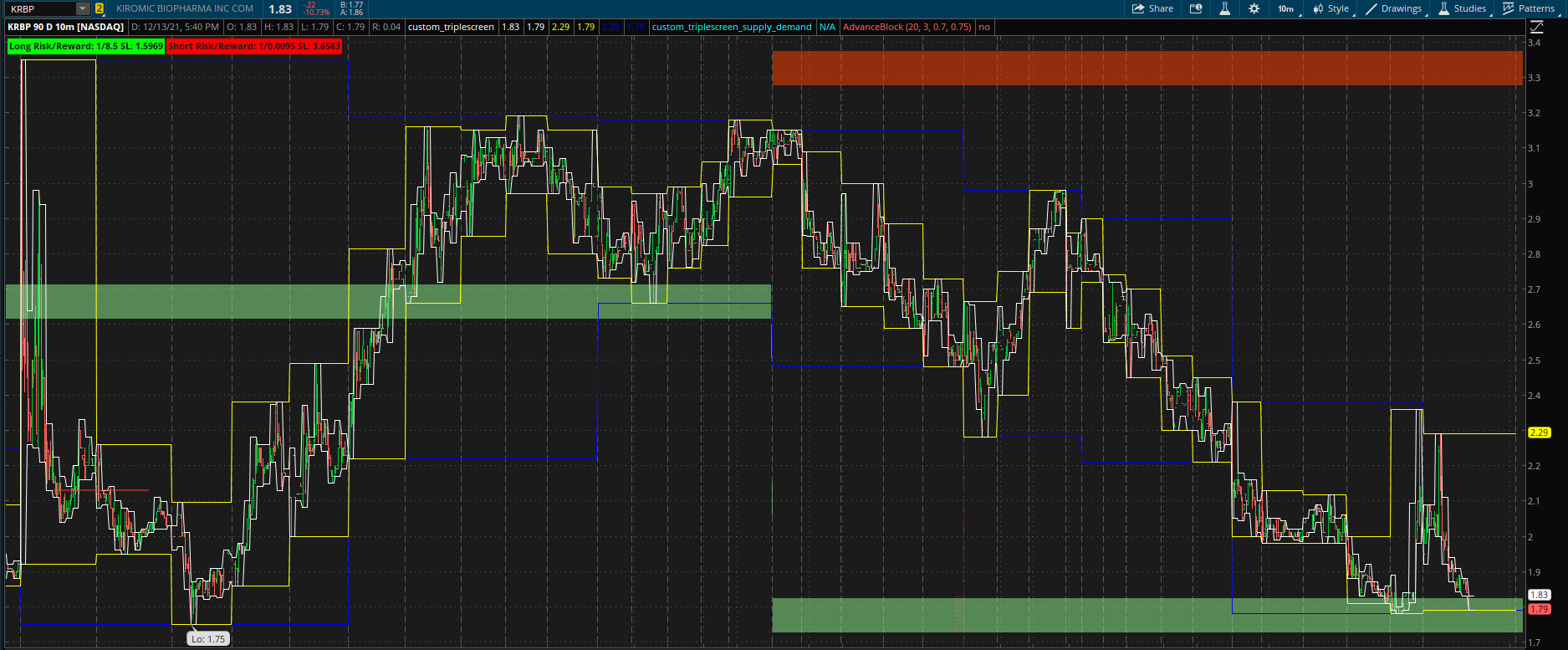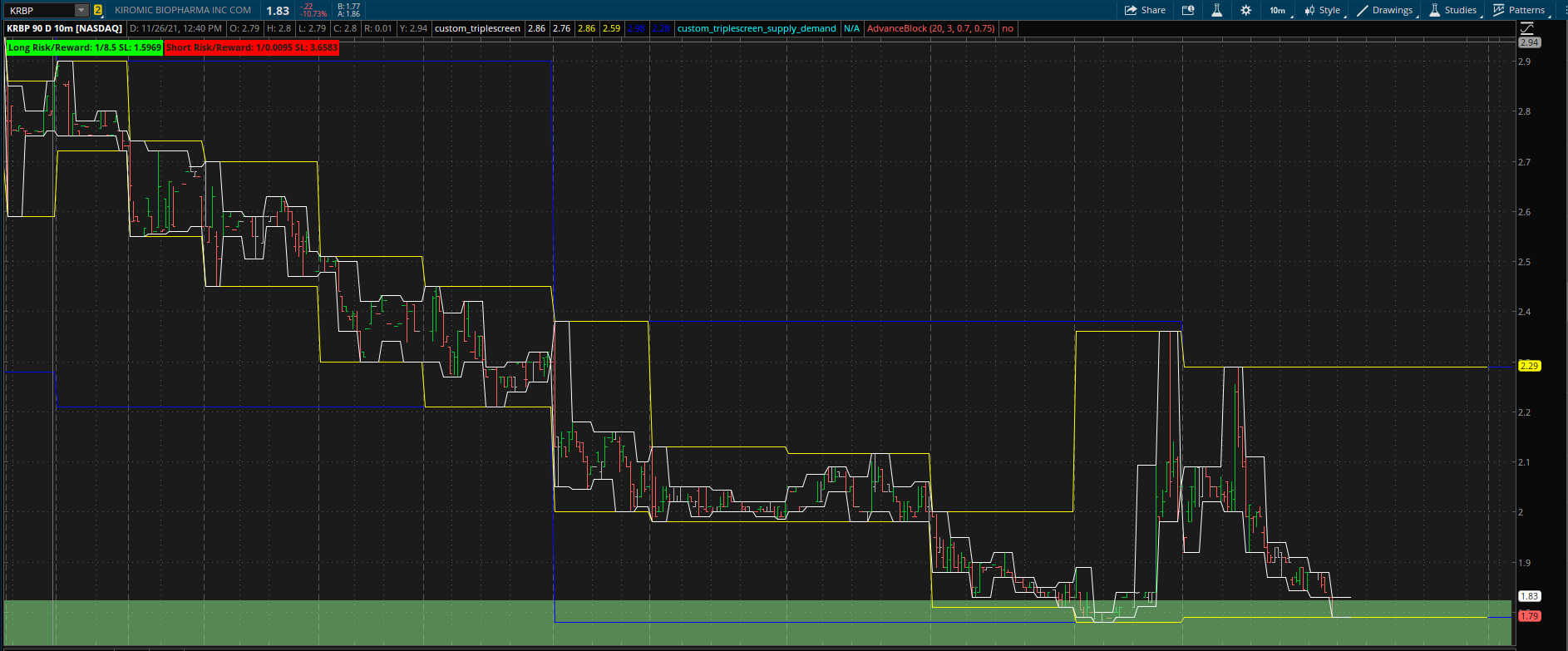# Triple Screen View For ThinkOrSwim

#### sid6.7

##### New member
Triple Screen View For ThinkOrSwim
I wanted to add multiple time frame bars into one screen, so I can see the movement of the stock. This is based on the idea of triple screen trading method. This setup was designed to work with 10min time frame. I used 90days of history you may want more, but it will run slower. Some stocks might not work well with this. This is just a visual aid and using other indicators along with it might help.

Legend:
The Red / Green zones are weekly pivots (I called it Supply/Demand but that isn't really the case). These act more like horizontal support and resistance from a weekly perspective.
The blue box is weekly high/low.
The yellow box is daily high/low.
The white box is hourly high/low.
The 10min candles are inner most.
The long risk/reward and short risk/reward are calculations for entering the stock at the current price with the goal of reaching the opposite red/green zones. Short red to green, Long green to red. This is also based on a 10% acceptable loss below or above the green / red zone. Stop Loss (SL) is stated in the label. Look for a green label and play that side of the market. When a green/red zone is breached beyond 10% an SL will have been hit, it does not suggest an entry. However, because these are basically weekly support/resistance, if the stock is bouncing below a green zone, it might be acting as resistance, you could use further analysis to determine if you want to take the trade to the downside. Same with Resistance acting as a support zone.

Weekly Pivot:
The prior 2 and future 2 weekly boxes (blue) need to be above, same, or below the current week to make a pivot. IE, red zone is 2 prior boxes and 2 future boxes are less than or equal to the high of my current week. If this does not happen, a red or green zone will not be created and will use the prior zones data. For some stocks like AAPL, the green zone might be dramatically further away from the red zone. For those stocks this indicator might not work well.

Repainting:
The support/resistance zones are calculated from the prior 2 weeks, so it will not repaint. The active week/day/hour, will repaint do to that period not yet closing. When an hour closes, it won't repaint, same for day and week.

Scanning:
There is no scanner, and unlikely to work if I tried because it does forward lookups to make the visual (I have not had much luck with forward lookups and scanning). What I do, is create other scans like RSI oversold in my price range (as an example), and then click on 1 of those and use the up/down arrow to scroll thru my list looking for viable trades.

How I use it:
My scanner is called Dollars, \$2 to \$10 with above 500k average volume. I look at the extremes by sorting using the "mark % change" column. I look for large price movements into a resistance and the same with support and analyze the trade further with volume and how the price reacts to these zones. Below is an example of what I look for with a long position. The spike in price when touching the green zone indicates demand to me, and the price has revisited allowing me to take the trade while keeping my risk low. The range is a 1:8 Risk/Reward, so I could do a 1:3 ratio and scale out 50% and close the trade if it falls back, or add to my position, etc. I have made multiple successful trades on both sides keeping my trading to 1:3 risk/reward no matter what the indicator tells me.Code:
``````#Sid6.7
#QUAD SCREEN w/ SUPPLY & DEMAND

def ap = GetAggregationPeriod();
def DOW = GetDayOfWeek(GetYYYYMMDD());
def cbar = BarNumber();
def lbar = HighestAll(if !IsNaN(close) then BarNumber() else 0);
def islastbar = cbar == lbar;
def lastbar = HighestAll(if IsNaN(close) then 0 else BarNumber());
def lastbaros = -(lastbar) + cbar;
def isfuture = lbar < BarNumber();
def islastbarofday = if islastbar or !IsNaN(DOW[-1]) and DOW[-1] != DOW then 1 else 0;
def isfirstbarofday = if !IsNaN(DOW) and DOW != DOW then 1 else 0;
def isfirstbarofweek = if DOW == 1 and isfirstbarofday then 1 else 0;
def islastbarofweek = if islastbar or DOW == 5 and islastbarofday then 1 else 0;
def endofweek = if DOW == 1 then 5
else if DOW == 2 then 4
else if DOW == 3 then 3
else if DOW == 4 then 2
else if DOW == 5 then 1
else 0;

def avgatr1 = ((Highest(ATR(), 500) + Lowest(ATR(), 500)) / 2);
def avgatr = GetValue(avgatr1, lastbaros);

#fast base = 1hr
def fastbase = AggregationPeriod.HOUR / ap;
#look forward to get candle count
def daycandlecount = if !isfirstbarofday then daycandlecount else
fold ci = 1 to AggregationPeriod.DAY / ap with cb = 1
while !IsNaN(GetValue(high, -ci)) and !GetValue(isfirstbarofday, -ci)
do cb + 1;
#trading day is 7hrs, so multiply fast base by 7
def normalbase = daycandlecount;
#look forward to get candle count
def weekcandlecount = if !isfirstbarofweek then weekcandlecount else
fold wi = 1 to AggregationPeriod.WEEK / ap with wb = 1
while !IsNaN(GetValue(high, -wi)) and !GetValue(isfirstbarofweek, -wi)
do wb + 1;
def slowbase =  weekcandlecount;

def cbarcount = if isfirstbarofday then 1 else cbarcount + 1;
def isfirstbarofhour = if isfirstbarofday or cbarcount % fastbase == 0 then 1 else 0;
def islastbarofhour = if islastbarofday or isfirstbarofhour[-1] then 1 else 0;

#look forward to get highs and lows of period
def fastbasehigh = if !isfirstbarofhour then fastbasehigh else
fold i = 0 to fastbase with b = 0
while i == 0 or (!IsNaN(GetValue(high, -i)) and !GetValue(isfirstbarofhour, -i))
do if GetValue(high, -i) > b then GetValue(high, -i) else b;

#fbh.setpaintingStrategy(paintingStrategy.HORIZONTAL);
def fastbaselow = if !isfirstbarofhour then fastbaselow else
fold fbli = 0 to fastbase with fblb = 10000
while fbli == 0 or (!IsNaN(GetValue(low, -fbli)) and !GetValue(isfirstbarofhour, -fbli))
do if GetValue(low, -fbli) < fblb then GetValue(low, -fbli) else fblb;

#fbl.setpaintingStrategy(paintingStrategy.HORIZONTAL);

plot fbh = fastbasehigh;
fbh.SetDefaultColor(Color.WHITE);
plot fbl = fastbaselow;
fbl.SetDefaultColor(Color.WHITE);

#look forward to get highs and lows of period
def normalbasehigh = if !isfirstbarofday then normalbasehigh else
fold dhi = 0 to normalbase with dhb = 0
while dhi == 0 or (!IsNaN(GetValue(high, -dhi)) and !GetValue(isfirstbarofday, -dhi))
do if GetValue(high, -dhi) > dhb then GetValue(high, -dhi) else dhb;
#fbh.setpaintingStrategy(paintingStrategy.HORIZONTAL);
def normalbaselow = if !isfirstbarofday then normalbaselow else
fold dli = 0 to normalbase with dlb = 10000
while dli == 0 or (!IsNaN(GetValue(low, -dli)) and !GetValue(isfirstbarofday, -dli))
do if GetValue(low, -dli) < dlb then GetValue(low, -dli) else dlb;
plot dbh = normalbasehigh;
dbh.SetDefaultColor(Color.YELLOW);
plot dll = normalbaselow;
dll.SetDefaultColor(Color.YELLOW);
#fbl.setpaintingStrategy(paintingStrategy.HORIZONTAL);

#look forward to get highs and lows of period
def slowbasehigh = if !isfirstbarofweek then slowbasehigh else
fold whi = 0 to slowbase with whb = 0
while whi == 0 or (!IsNaN(GetValue(high, -whi)) and !GetValue(isfirstbarofweek, -whi))
do if GetValue(high, -whi) > whb then GetValue(high, -whi) else whb;
#fbh.setpaintingStrategy(paintingStrategy.HORIZONTAL);
def slowbaselow = if !isfirstbarofweek then slowbaselow else
fold wli = 0 to slowbase with wlb = 10000
while wli == 0 or (!IsNaN(GetValue(low, -wli)) and !GetValue(isfirstbarofweek, -wli))
do if GetValue(low, -wli) < wlb then GetValue(low, -wli) else wlb;
plot wbh = slowbasehigh;
wbh.SetDefaultColor(Color.BLUE);
plot wll = slowbaselow;
wll.SetDefaultColor(Color.BLUE);
#fbl.setpaintingStrategy(paintingStrategy.HORIZONTAL);

#weekly supply & demand
#look forward 2 weeks and look back 2 weeks and make sure my low is then same or lower
def oneweek = if isfirstbarofweek then (fold owi = 1 to 500 with owb = 0
while owb == 0
do if GetValue(isfirstbarofweek, owi) then GetValue(cbar, owi) else owb) else oneweek;
def onefutweek = if isfirstbarofweek then (fold owi2 = 1 to 500 with owb2 = 0
while owb2 == 0 and !IsNaN(GetValue(close, -owi2))
do if GetValue(isfirstbarofweek, -owi2) then GetValue(cbar, -owi2) else owb2) else onefutweek;
def twoweeks = GetValue(oneweek, (cbar - oneweek));
def twofutweek = GetValue(onefutweek, cbar - onefutweek);

def isweekpeak = GetValue(wbh, cbar - twoweeks) <= wbh and GetValue(wbh, cbar - oneweek) <= wbh and GetValue(wbh, cbar - onefutweek) <= wbh and GetValue(wbh, cbar - twofutweek) <= wbh;
def isweekspike = GetValue(wll, cbar - twoweeks) >= wll and GetValue(wll, cbar - oneweek) >= wll and GetValue(wll, cbar - onefutweek) >= wll and GetValue(wll, cbar - twofutweek) >= wll;

def weeklymidsupply = if IsNaN(isweekpeak) then weeklymidsupply else if isweekpeak and islastbarofweek then (wbh - (avgatr / 2)) else weeklymidsupply;
def a1 = GetValue(weeklymidsupply, cbar - twoweeks);
AddCloud(a1 + avgatr, a1 - avgatr, Color.LIGHT_RED, Color.LIGHT_RED);
#look back 2 weeks and see if supply pivot

def weeklymiddemand = if IsNaN(isweekspike) then weeklymiddemand else if isweekspike and islastbarofweek then (wll + (avgatr / 2))  else weeklymiddemand;
def a2 = GetValue(weeklymiddemand, cbar - twoweeks);
AddCloud(a2 + avgatr, a2 - avgatr, Color.LIGHT_GREEN, Color.LIGHT_GREEN);

#10% below green SL, in red TP
def bullrisk = ceil((((a2-(a2*.10)) - close)/close) * 100);
def bullreward = ceil(((close - (a1))/close) * 100);
def bullratio = (bullreward / bullrisk);
addlabel(bullratio > 2,"Long Risk/Reward: 1/"+ bullratio + " SL: " + (a2-(a2*.10)), color.green);
addlabel(bullratio > 1 and bullratio <= 2,"Long Risk/Reward: 1/"+ bullratio + " SL: " + (a2-(a2*.10)), color.yellow);
addlabel(bullratio <= 1,"Long Risk/Reward: 1/"+ bullratio + " SL: " + (a2-(a2*.10)));

def bearrisk = ceil((((a1+(a1*.10)) - close)/close) * 100);
def bearreward = ceil(((close - (a2))/close) * 100);
def bearratio = (bearreward / bearrisk);
addlabel(bearratio > 2,"Short Risk/Reward: 1/"+ bearratio + " SL: " + (a1+(a1*.10)), color.green);
addlabel(bearratio > 1 and bearratio <= 2,"Short Risk/Reward: 1/"+ bearratio + " SL: " + (a1+(a1*.10)), color.yellow);
addlabel(bearratio <= 1,"Short Risk/Reward: 1/"+ bearratio + " SL: " + (a1+(a1*.10)));

Last edited by a moderator:
••ApeX Predator and MerryDay
when I run this study chart was shrink what is the reason

when I run this study chart was shrink what is the reason
right click the chart, and hover chart scale, and untoggle "fit studies". The red and green lines are created on weekly pivots. So, if it has a dramatic or long term downtrend, the distance between the green and red pivot would be substantial and crunch the chart if "fit studies" was toggled, because it will try to fit the chart with the red and green bar.

•MerryDay

### Not the exact question you're looking for?

87k+ Posts
355 Online## The Market Trading Game Changer

Join 2,500+ subscribers inside the useThinkScript VIP Membership Club
• Exclusive indicators
• Proven strategies & setups
• Private Discord community
• Exclusive members-only content
• 1 full year of unlimited support

What is useThinkScript?

useThinkScript is the #1 community of stock market investors using indicators and other tools to power their trading strategies. Traders of all skill levels use our forums to learn about scripting and indicators, help each other, and discover new ways to gain an edge in the markets.

How do I get started?

We get it. Our forum can be intimidating, if not overwhelming. With thousands of topics, tens of thousands of posts, our community has created an incredibly deep knowledge base for stock traders. No one can ever exhaust every resource provided on our site.

If you are new, or just looking for guidance, here are some helpful links to get you started.

What are the benefits of VIP Membership?